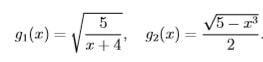# CS 261/MATH 261 Methods of Numerical Analysis

## Assignment 3

(8 marks) Let the functions g1 and g2 be defined by• Show that 1 is a fixed point of g1 and g2, and that any fixed point of g1 or g2 is a root of the equation x3 + 4x2 − 5 = 0.
• Suppose that we take x0 sufficiently close to 1 (but not equal to 1) and would like to generate a sequence {xn} by the fixed-point iteration xn = g1(xn−1) (n ≥ 1) or by the fixed-point iteration xn = g2(xn−1) (n ≥ 1). If you wish to have faster convergence (to the point 1), which of the two fixed-point iterations would you choose? Justify your answer. (You need to make your choice by a theoretical result, not by comparing the first few iterates.)
1. (6 marks) Let g(x) = (2x2 + 3)1/4.
• Let p0 = 1. Find p10 by the fixed-point iteration

pn+1 = g(pn) (n ≥ 0).

(You should use MATLAB to do this. But just report p10 with at least 10 digits. )

• Let = 1. Use Steffensen’s method to find and. (You should use MATLAB to do this. But just report and, with at least 10 digits. )
• Show that √3 is a fixed point of g. Which of p10 and gives a better approximation to this fixed point?
1. (6 marks) Let f(x) = x4 + 3x3 − 4x2 + 3x − 2.

Find f(1.6) and f(1.6) by Horner’s method using a table form (with the help of a calculator).

1. (5 marks) Let f(x) = x3 x−1 and x0 = 1.1,x1 = 1.2,x2 = 1.3 be approximations to a solution of f(x) = 0. Use Mu¨ller’s method to find the next approximation x3.
2. (5 marks) Suppose that Newton’s method is applied to find the solution p = 0 of the equation = 0. It is known that, starting with any p0 > 0, the sequence {pn} produced by the Newton’s method is monotonically decreasing (i.e., p0 > p1 > p2 > ··) and converges to 0.

Prove that {pn} converges to 0 linearly with rate 2/3. (hint: You need to have the patience to use L’Hospital rule repeatedly. )